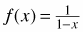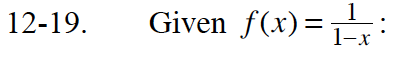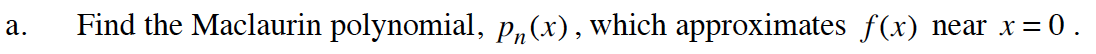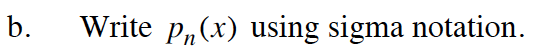### Home > CALC > Chapter 12 > Lesson 12.1.2 > Problem12-19

12-19.
1. Given: Homework Help ✎

1. Find the Maclaurin polynomial, pn (x), which approximates f(x) near x = 0.

2. Write pn (x) using sigma notation.The original function and first few derivatives are listed below. Do you see a pattern?

Evaluate the original function and each of the derivatives at x = 0.Simplify the terms in the equation given in Step 2 (a). Then write an expression for the general term. Use this in your summation.